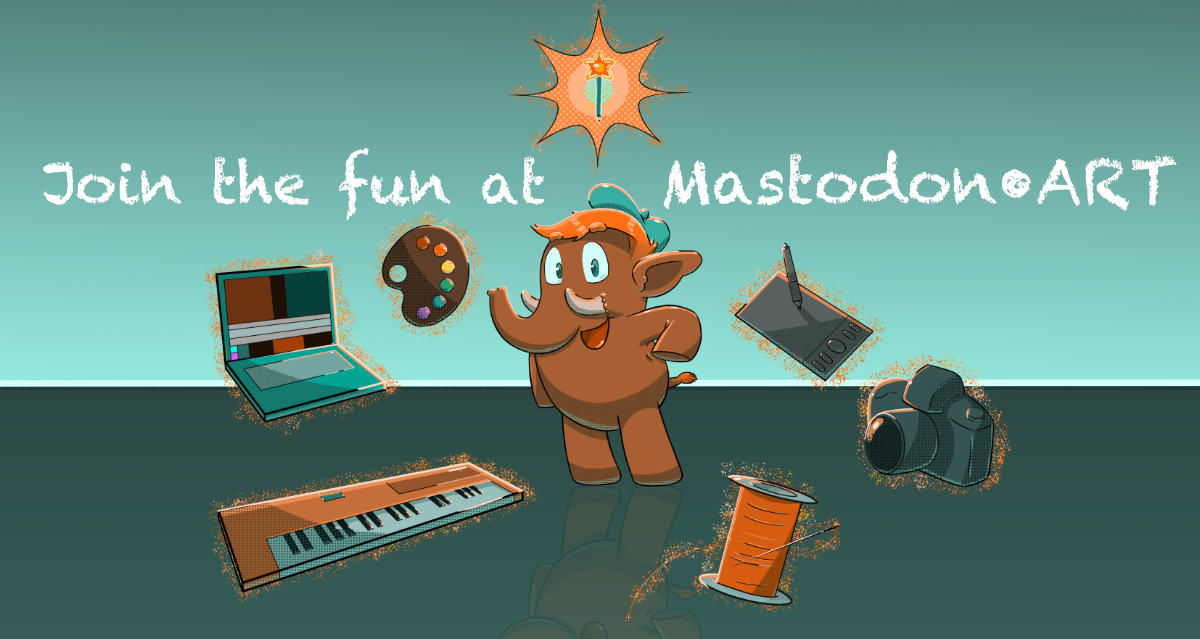Hand Cram!
A game that fits into just 2 tweets (560 chars). It *almost* fits in a single toot.

Arrows control white, ESDF controls red.
If the two circles touch, you lose.
Hitting opposite color dots grows the circle

Src in replies

Play it here: andymakes.itch.io/hand-cram

· · Web · · ·

t=0g=0r=rnd
w=128b=abs
o=circ::_::if g>0then
pal({5,2},1)print("score:"..t,9,9,7)
if(btn(5))t,g=0,0
else
if(t<1)a={9,9,88,0,40,0,0,0,0,0,40,0,88}
i=btn()pal({7,8},1)t+=1
for k=0,200do
o(r(w),r(w),5,0)end
for k=0,10do
s=2^k
l=s*2
a[k+3]+=band(i,l)/l-band(i,s)/s
end
for k=0,1do
x=a[3+k*8]y=a[5+k*8]s=a[k+1]o(x,y,s,1+k)o(x,y,s+2,1+k)
for c=0,w do
srand(c+t)if r(8)<1then
p=1+flr(r(2))z=r(w)o(c,z,4,p)
if(sqrt((x-c)^2+(y-z)^2)<s)a[k+1]+=b(1+k-p)/5-.01end
end

if(k==1 and sqrt((x-a)^2+(y-a)^2)<s+a)g=1
if(b(64-x)>66or 66<b(64-y))g=1end
end
flip()goto _

If you are curious about how this code works, I wrote a highly commented version of it here: gist.github.com/andymasteroffi

The actual code is identical, but I broke it up with whitespace, line breaks a ton of comments describing what each part does

(it also probably has a lot of typos)Mastodon.ART — Your friendly creative home on the Fediverse! Interact with friends and discover new ones, all on a platform that is community-owned and ad-free. Admin: @Curator. Moderators: @EmergencyBattle, @ScribbleAddict, @TapiocaPearl, @Otherbuttons, @katwylder